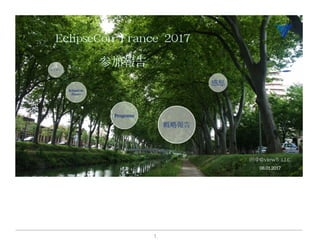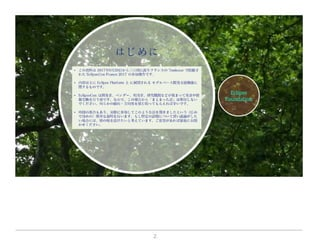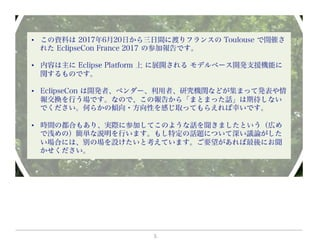Successfully reported this slideshow.

# Eclipse con2017参加報告公開版

3

Share×
1 of 121
1 of 121

# Eclipse con2017参加報告公開版

3

Share

Presented at MBSD Community gathering on Aug. 1, 2017.

Presented at MBSD Community gathering on Aug. 1, 2017.

## More Related Content

### Related Books

Free with a 14 day trial from Scribd

See all

### Related Audiobooks

Free with a 14 day trial from Scribd

See all

1. 1. 1.
2. 2. 2.
3. 3. 3.
4. 4. 4.
5. 5. 5.
6. 6. 6.
7. 7. 7.
8. 8. 8.
9. 9. 9.
10. 10. 10.
11. 11. 11.
12. 12. 12.
13. 13. 13.
14. 14. 14.
15. 15. 15.
16. 16. 16.
17. 17. 17.
18. 18. 18.
19. 19. 19.
20. 20. 20.
21. 21. 21.
22. 22. 22.
23. 23. 23.
24. 24. 24.
25. 25. 25.
26. 26. 26.
27. 27. 27.
28. 28. 28.
29. 29. 29.
30. 30. 30.
31. 31. 31.
32. 32. 32.
33. 33. 33.
34. 34. 34.
35. 35. 35.
36. 36. 36.
37. 37. 37.
38. 38. 38.
39. 39. 39.
40. 40. 40.
41. 41. 41.
42. 42. 42.
43. 43. 43.
44. 44. 44.
45. 45. 45.
46. 46. 46.
47. 47. 47.
48. 48. 48.
49. 49. 49.
50. 50. 50.
51. 51. 51.
52. 52. 52.
53. 53. 53.
54. 54. 54.
55. 55. 55.
56. 56. 56.
57. 57. 57.
58. 58. 58.
59. 59. 59.
60. 60. 60.
61. 61. 61.
62. 62. 62.
63. 63. 63.
64. 64. 64.
65. 65. 65.
66. 66. 66.
67. 67. 67.
68. 68. 68.
69. 69. 69.
70. 70. 70.
71. 71. 71.
72. 72. 72.
73. 73. 73.
74. 74. 74.
75. 75. 75.
76. 76. 76.
77. 77. 77.
78. 78. 78.
79. 79. 79.
80. 80. 80.
81. 81. 81.
82. 82. 82.
83. 83. 83.
84. 84. 84.
85. 85. 85.
86. 86. 86.
87. 87. 87.
88. 88. 88.
89. 89. 89.
90. 90. 90.
91. 91. 91.
92. 92. 92.
93. 93. 93.
94. 94. 94.
95. 95. 95.
96. 96. 96.
97. 97. 97.
98. 98. 98.
99. 99. 99.
100. 100. 100.
101. 101. 101.
102. 102. 102.
103. 103. 103.
104. 104. 104.
105. 105. 105.
106. 106. 106.
107. 107. 107.
108. 108. 108.
109. 109. 109.
110. 110. 110.
111. 111. 111.
112. 112. 112.
113. 113. 113.
114. 114. 114.
115. 115. 115.
116. 116. 116.
117. 117. 117.
118. 118. 118.
119. 119. 119.
120. 120. 120.
121. 121. 121.